# NCERT Solutions for Class 9 Maths chapter 1 Exercise 1.3 - Number System - Free PDF Download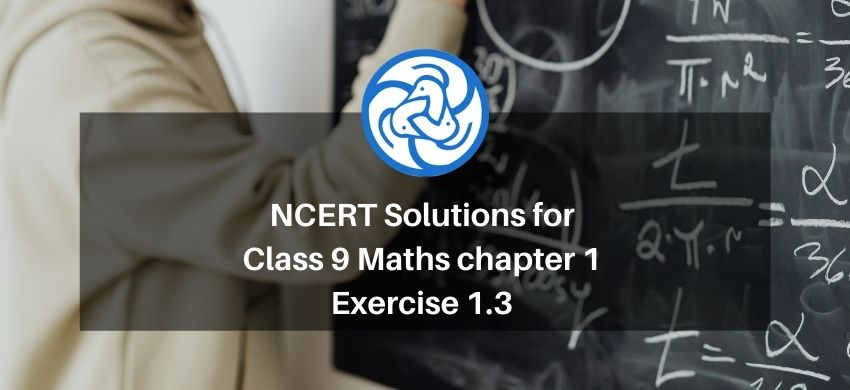Hey, are you a class 9 Student and Looking for Ways to Download NCERT Solutions for Class 9 Maths chapter 1 Exercise 1.3? If Yes then you are at the right place.

Here we have listed Class 9 maths chapter 1 exercise 1.3 solutions  in PDF that is prepared by Kota’s top IITian’s Faculties by keeping Simplicity in mind.

If you want to score high in your class 9 Maths Exam then it is very important for you to have a good knowledge of all the important topics, so to learn and practice those topics you can use eSaral NCERT Solutions.

So, without wasting more time Let’s start.

### Download The PDF of NCERT Solutions for Class 9 Maths chapter 1 Exercise 1.3 "Number System"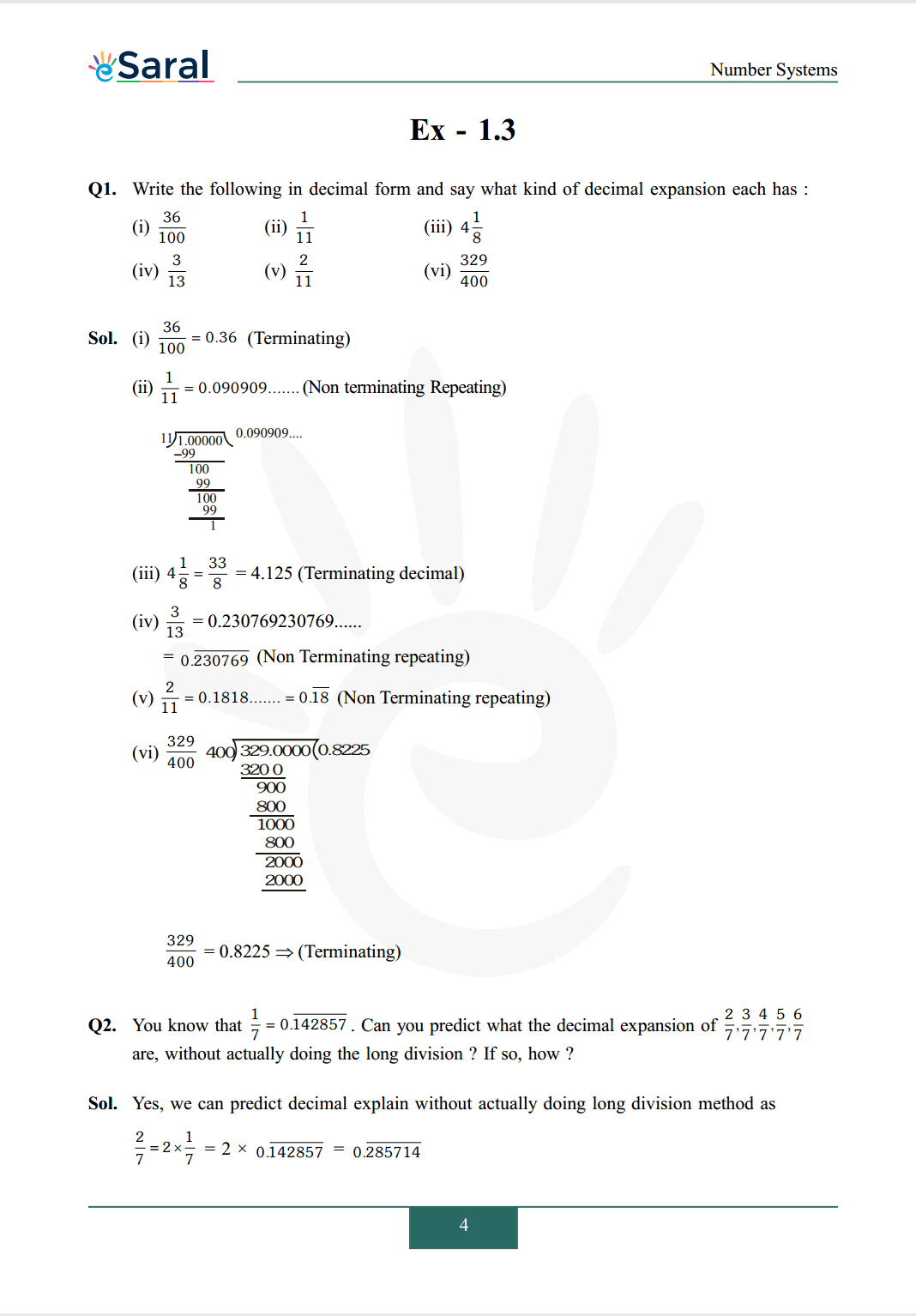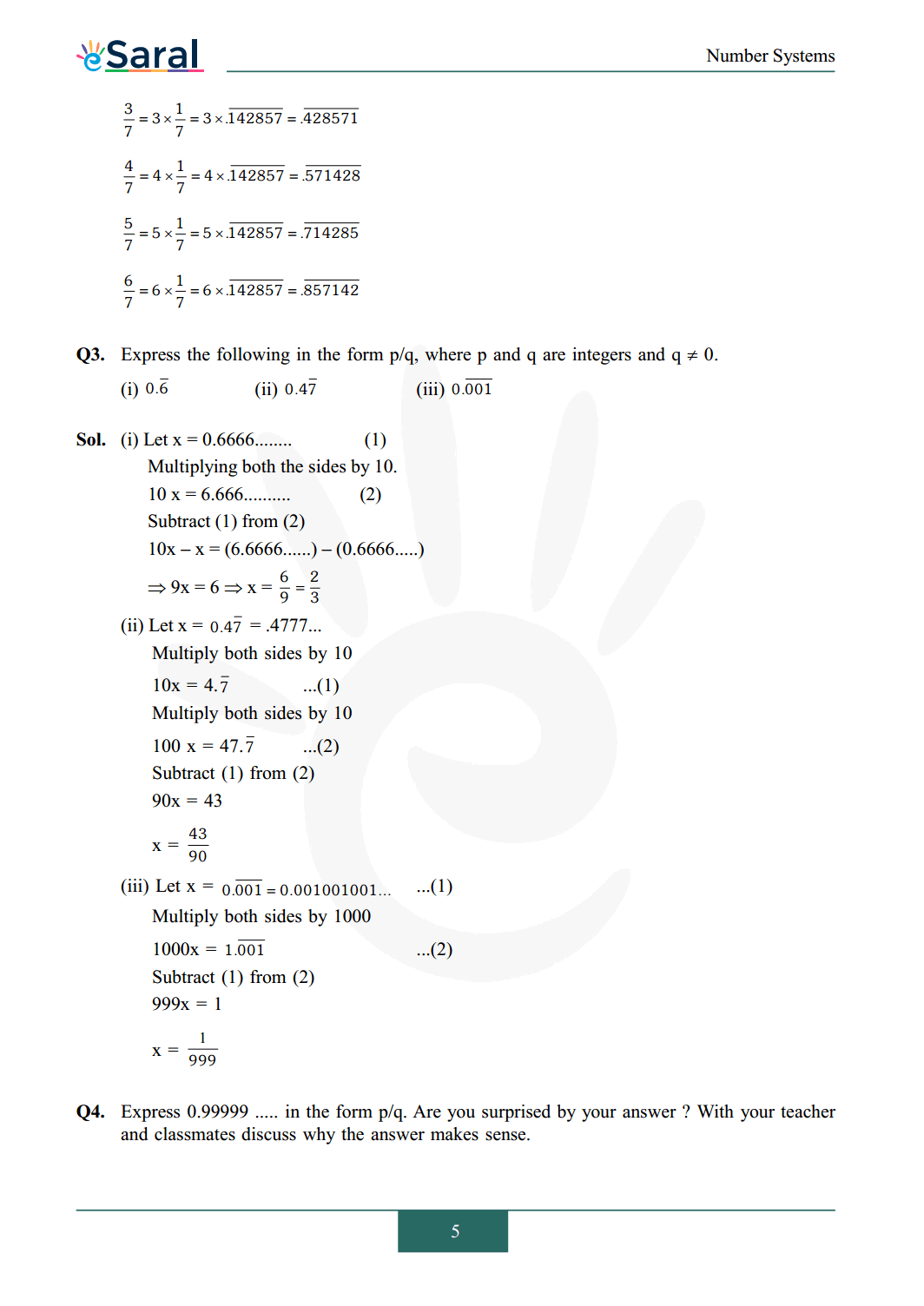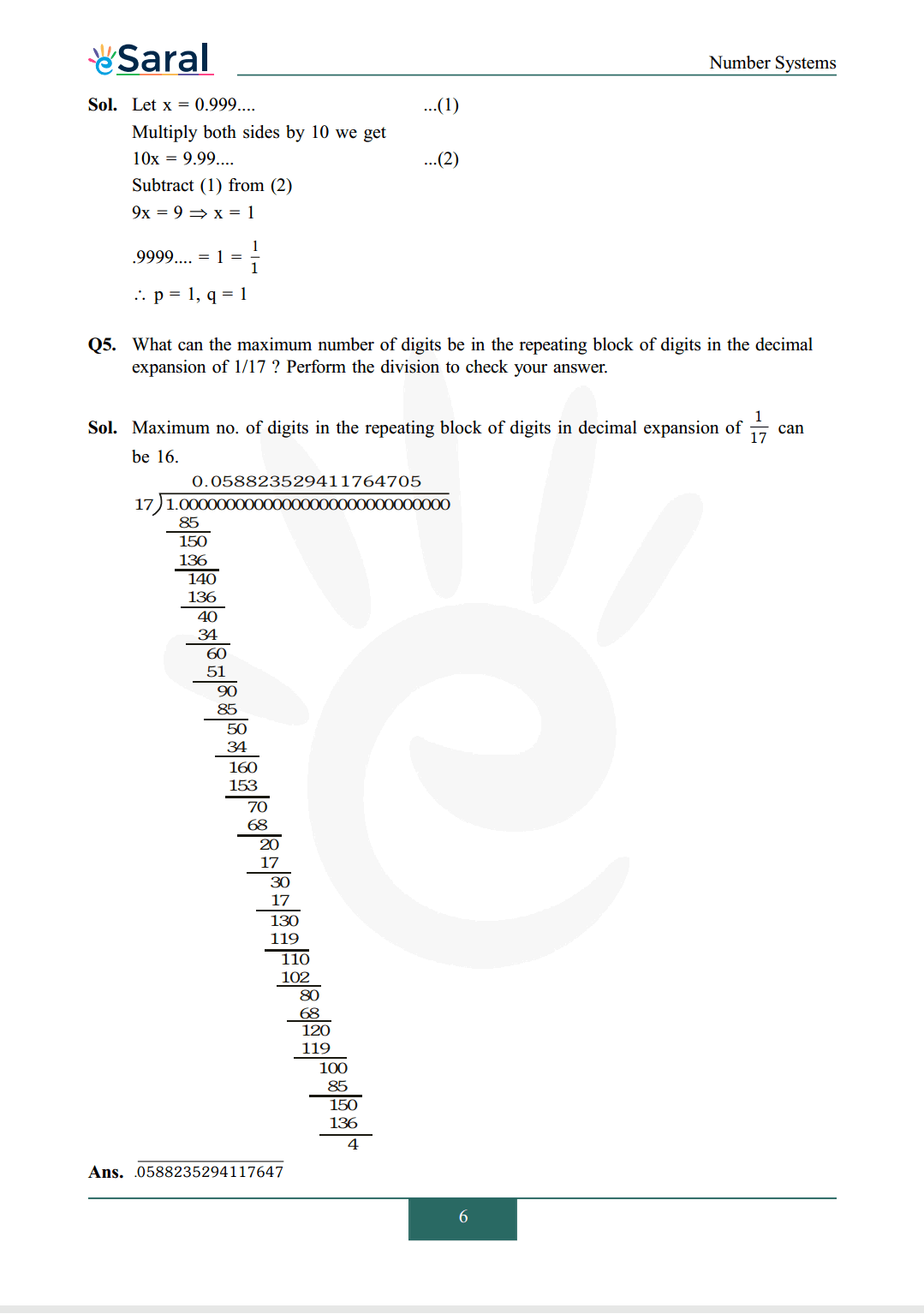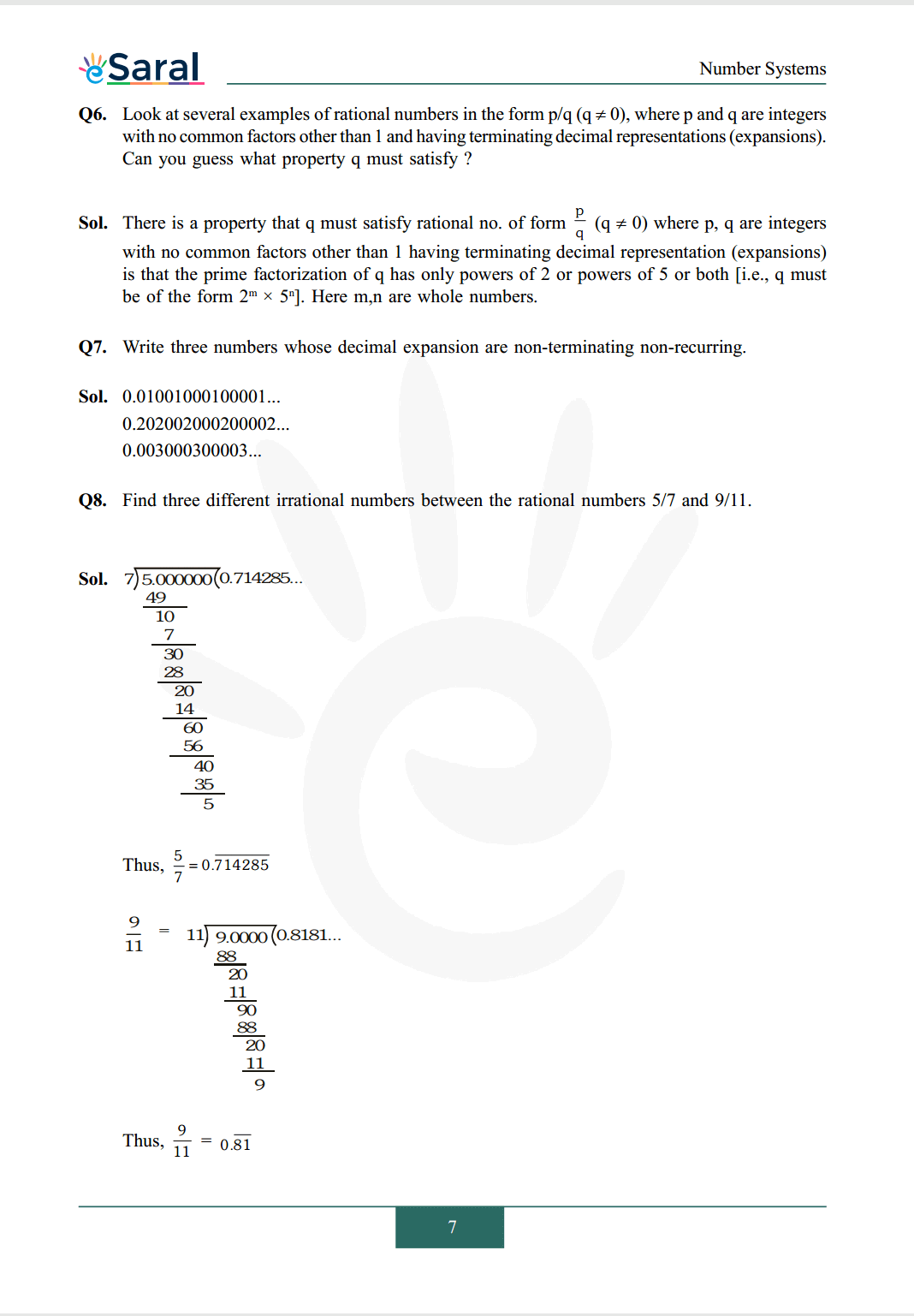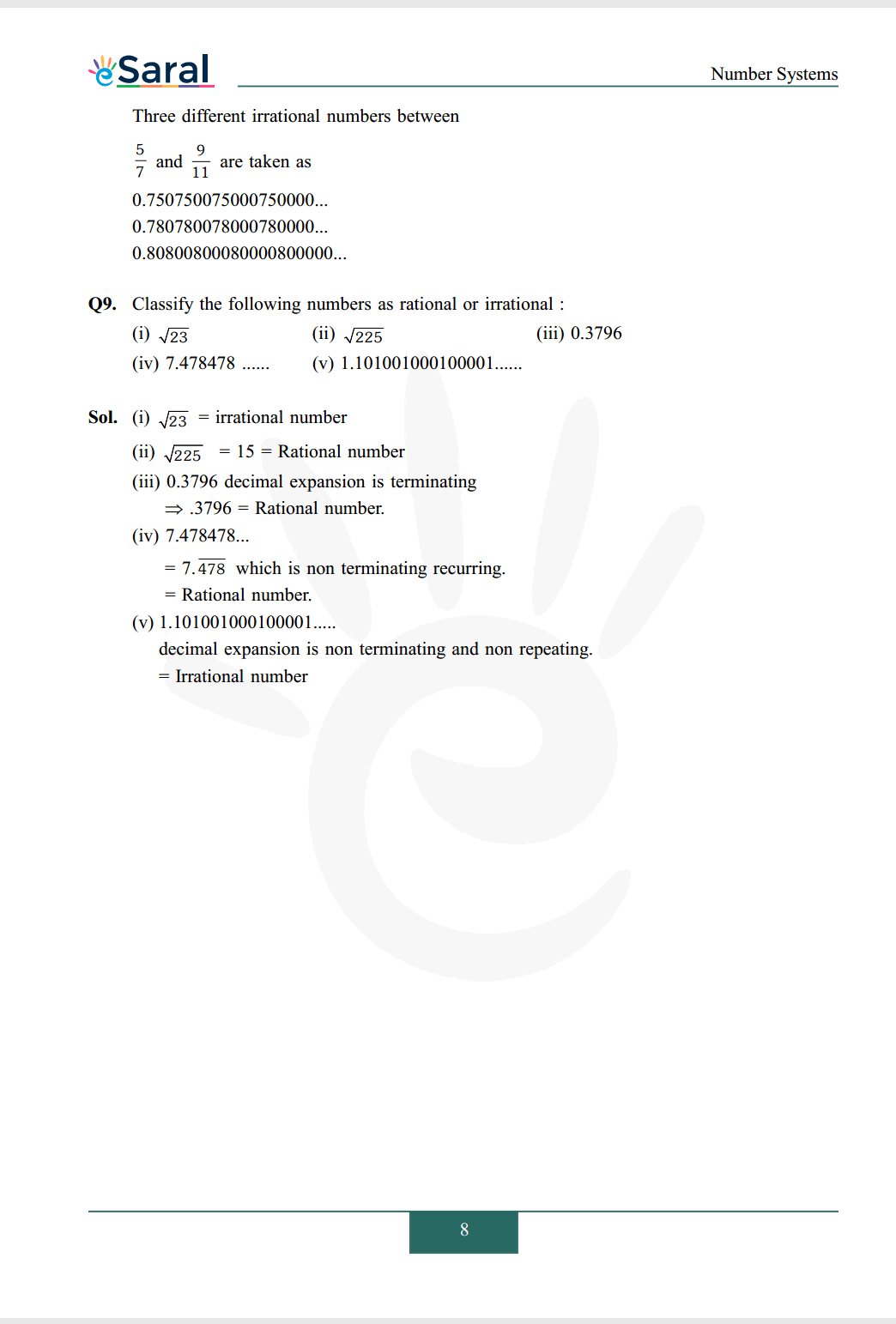#### All Questions of Chapter 1 Exercise 1.3

Once you complete the chapter 1 then you can revise Ex. 1.3 by solving following questions

Q1. Write the following in decimal form and say what kind of decimal expansion each has :
(i) $\frac{36}{100}$
(ii) $\frac{1}{11}$
(iii) $4 \frac{1}{8}$
(iv) $\frac{3}{13}$
(v) $\frac{2}{11}$
(vi) $\frac{329}{400}$

Q2. You know that $\frac{1}{7}=0 . \overline{142857}$. Can you predict what the decimal expansion of $\frac{2}{7}, \frac{3}{7}, \frac{4}{7}, \frac{5}{7}, \frac{6}{7}$ are, without actually doing the long division ? If so, how ?

Q3. Express the following in the form $\mathrm{p} / \mathrm{q}$, where $\mathrm{p}$ and $\mathrm{q}$ are integers and $\mathrm{q} \neq 0$.
(i) $0 . \overline{6}$
(ii) $0.4 \overline{7}$
(iii) $0 . \overline{001}$

Q4. Express $0.99999 \ldots \ldots$ in the form p/q. Are you surprised by your answer ? With your teacher and classmates discuss why the answer makes sense.

Q5. What can the maximum number of digits be in the repeating block of digits in the decimal expansion of $1 / 17$ ? Perform the division to check your answer.

Q6. Look at several examples of rational numbers in the form $\mathrm{p} / \mathrm{q}(\mathrm{q} \neq 0)$, where $\mathrm{p}$ and $\mathrm{q}$ are integers with no common factors other than 1 and having terminating decimal representations (expansions). Can you guess what property q must satisfy ?

Q7. Write three numbers whose decimal expansion are non-terminating non-recurring.

Q8. Find three different irrational numbers between the rational numbers $5 / 7$ and $9 / 11$.

Q9. Classify the following numbers as rational or irrational :
(i) $\sqrt{23}$
(ii) $\sqrt{225}$
(iii) $0.3796$
(iv) $7.478478 \ldots \ldots$
(v) $1.101001000100001 \ldots \ldots$

Class 9 Maths Revision Notes.